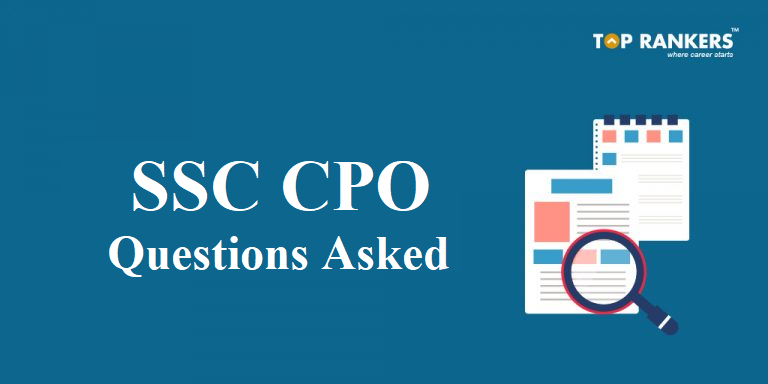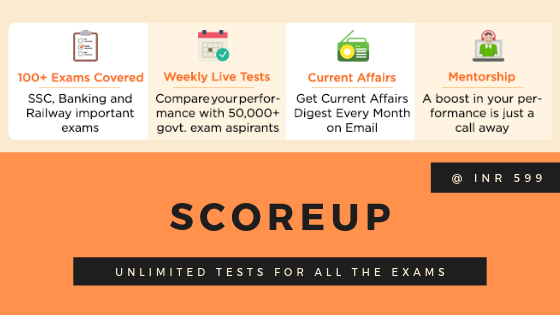SSC CPO Questions Asked:- As SSC conducted CPO examination from 12th to 16th March 2019. Candidates who are preparing for the next segment of SSC Central Police Organisation, CPO are looking for questions asked in the examination.

• SSC conducts CPO examination for recruitment of Sub-inspector (SI) for organizations such as CRPF, ITBP, CBI, SSB, CISF, BSF etc.
• Find here, video on SSC CPO Questions asked in SSC CPO 2018 examination which was conducted from 12th March to 16th March 2019.
• Exam Analysis can be another great material to prepare for SSC CPO, read our detailed SSC CPO Exam Analysis for All day and All shifts
• We have also brought for you, SSC CPO Questions asked PDF (Mathematics)
• Find detailed video solution for the questions asked in SSC CPO  exam.
• Aspirants who are preparing for SSC CPO 2019 can get an insight of the type of questions asked in Central Police Organization, CPO conducted by SSC.Questions asked in SSC CPO 2018-19 exam will help you make a plan for smooth exam preparation. watch the video mentioned-below to know detailed solution and best tricks and tricks;

#### Math Questions – SSC CPO Questions Asked

Find solutions of the SSC CPO Questions asked in the 2018-19 examination conducted from 12th March to 16th March 2019;

### Questions Paper PDF – SSC CPO questions asked (12th march to 16th March 2019)

1. If a3 – b3 = 208, a – b = 4 then = (a + b)2 – ab = ?
2. (x – 7)3 + (x – 6)3 + (x – 5)3 = 3 (x – 7) (x – 6) (x – 5) find x = ?
3. If r = 1 find the area of shaded region?
4. ABCD is cyclic, trapezium AB diameter ÐADC = 140 find ÐBAC = ?
5. Find the area of rhombus where side is 5 cm & one diagonal is 8 cm.
6. Average of 11 player is 20 two new player of age 25 & 15 has join them find the new average?
7. tan 2 q = cat (30 + 2 q) then q = ?
8. Five cubes of side 4 cm are fixed together find the total surface area of the new solid formed?
9. If the radius of a cylinder is decreased by 50% and height is increased by 50% then what is change in volume?
10. Two articles are sold at same price one at a profit of 13% and other one at loss of 13% what is overall profit or loss%?
11. If (2xy)2 + (3y – 2z)2 = 0 then x : y : z = ?
12. Four cubes are melted together to form big cube. Volume of larger cube 6912 cm3 find the total surface area of each smaller cube?
13. cos q =  then tan q ?
14. If principal amount is Rs. 2000 and rate of interest is 10% compounded half yearly then find the compound interest for 15 month?
15. Find the equivalent discount of 15%, 20%, 37.5% = ?
16. The price of sugar is reduced by 25% then find increase in consumption so that there is no effect on expenditure?
17. x, y can complete a work in 10 days and 15 days respectively working individually. Both start the work together and after 3 days x left the work in how many days, y completes the reaming work.
18. A & B can do a work in 6 days and 9 days working alone. In how many days they can do 35% of whole work. If they work on alternate days starting with A.
19. Two pipes can fill a tank in 6 hrs. & 9 hrs. and a third pipe empty tank in 12 hrs. then 45% of tank fill in how much time?

Check SSC CPO Exam Analysis and Questions Asked – Shift 1 – All Day

Check SSC CPO Exam Analysis and Questions Asked – Shift 2 – All Day

In the PDF below, we have mentioned questions asked along with diagrams in SSC CPO Math examination conducted recently;Hope you liked this post on SSC CPO Questions asked. If you have any query or suggestion, do write to us in the comments below.